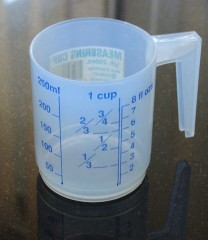# How Many 1/3 Cups Equals 1 Cup?Three 1/3 cups would equal one cup.

One third of a cup equals five tablespoons, plus one teaspoon. A tablespoon is equivalent to three teaspoons, so one third of a cup contains 16 teaspoons. As one cup equals 48 teaspoons, three 1/3 cups would add up to the equivalent of a cup.

These kinds of measurements are most commonly used in cooking and recipes. There are 8 ounces in one cup, so in a third of a cup, there is 2.66 ounces.

A cup of butter generally weighs about 8 ounces. 1 ounce is the equivalent of 28.34 grams, so one cup of butter weighs around 227 grams.

A third of a cup of all-purpose flour is equal to 43 grams or 1.5 ounces. A third of a cup of rolled oats is equivalent to 28 grams and 1 ounce. A third of a cup of white sugar is equivalent to 67 grams and 2.37 ounces. A third of a cup of honey or syrup is equivalent to 113 grams or four ounces.

A third of a cup of milk is equivalent to 75 millilitres. If you need to cut a recipe by a third, then use a third of a cup measurements. For example a third of a cup of butter will weigh approximately 79 grams.
thanked the writer.